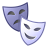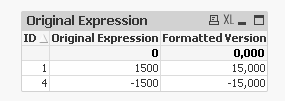Announcements
You can succeed best and quickest by helping others to succeed. Join the conversation.
cancel
Showing results for
Did you mean:Contributor

## Number Ranges

Trying to extract below number ranges:

- Amount larger than 1000

AND

- Amount smaller than -1000

Below is my expression:

num(sum({<[Ledger Type]={'AA'},Source={'F0911'},Amount={">1000<-1000"}>} Amount/100),'#,##0')

However it is still pulling in zeros and numbers smaller than 1000 and larger than 1000.

Can anyone help on how to fix it?

Thanks

1 Solution

Accepted SolutionsCreator II

Hi Richard,

Try this -

Sum({<Amount= {">1000"},[Ledger Type]={'AA'},Source={'F0911'}>

+

<Amount= {"<-1000"},[Ledger Type]={'AA'},Source={'F0911'}>

}Amount)

Thanks,

RT

5 RepliesPartner - Specialist

Hi,

Made a table with following inline table:

Figures:

ID, Value

1, 1500

2, 999

3, -999

4, -1500

];

And used this expression:

Sum({<Value = {">=1000<=-1000"}>}Value)

num(Sum({<Value = {">=1000<=-1000"}>}Value / 100),'#,##0')

And that gave me:Is this what you are trying to do?

Best,

Ali APartner - Specialist

Also,

If you could add some data that you have could be easier to try with that data...Contributor
Author

Thanks Ali.

Other than using the inline method, is there a way to simply use set analysis to return the data that I need?

If I use the Amount={">1000"}, it gives me correct data that larger than 1,000.

Likewise, Amount={"<-1000"} returns anything less than -1,000.

The problem is when I combine them into Amount={">10000000<-10000000"} which returns inconsistent data.Creator II

Hi Richard,

Try this -

Sum({<Amount= {">1000"},[Ledger Type]={'AA'},Source={'F0911'}>

+

<Amount= {"<-1000"},[Ledger Type]={'AA'},Source={'F0911'}>

}Amount)

Thanks,

RTContributor
Author

Thanks Rajesh that works.Tags
Community Browser